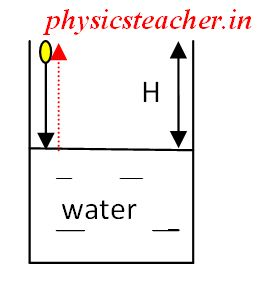# Solution to problems – class 9 – Set 1 Q47

Last updated on June 17th, 2019 at 02:47 pm

## Problem Statement

47) A pebble is dropped freely in a well from its top. It takes 20 s for the pebble to reach the water surface in the well. Taking g = 10 m/s^2 and speed of sound = 330 m/s, find: (i) the depth of water surface, and (ii) the time when echo is heard after the pebble is dropped.

## Solutionpebble (yellow) is falling – shown as downward arrow, The red upward line shows traversal of sound wave

Say, the depth of water surface in the well
= the distance between the point where from the pebble is released and the surface of water in the well = H

It takes 20 s for the pebble to reach the water surface in the well. So,  t = 20 sec

From equation, H = ut + (½) g t2

As u is 0 (released from rest, assumed), the equation becomes H = (½) g t2
So,we get H= ½ . 10. 202 = 2000 m……..(answer of i)

The time when echo is heard after the pebble is dropped =

time required by the pebble to touch the water surface after being released + the time required by the sound wave generated to traverse the height of 2000 m to reach the person

= 20 sec + 2000/330 sec
= (20 + 6.06) sec= 26.06 seconds…………..(answer of ii)

Question Set – Physics Problems for grade 9

See also  Solution to problems - class 9 - Set 1 Q31, Q32, Q33
Scroll to top
error: physicsTeacher.in